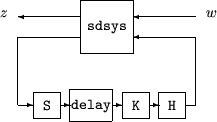# sdhinfnorm

Compute L2 norm of continuous-time system in feedback with discrete-time system

## Syntax

```[gaml,gamu] = sdhinfnorm(sdsys,k)
[gaml,gamu] = sdhinfnorm(sdsys,k,delay)
[gaml,gamu] = sdhinfnorm(sdsys,k,delay,tol)
```

## Description

`[gaml,gamu] = sdhinfnorm(sdsys,k) ` computes the L2 induced norm of a continuous-time LTI plant, `sdsys`, in feedback with a discrete-time controller, `k`, connected through an ideal sampler and a zero-order hold (see figure below). `sdsys` must be strictly proper, such that the constant feedback gain must be zero. The outputs, `gamu` and `gaml`, are upper and lower bounds on the induced L2 norm of the sampled-data closed-loop system.`[gaml,gamu] = sdhinfnorm(sdsys,k,h,delay) ` includes the input argument `delay`. `delay` is a nonnegative integer associated with the number of computational delays of the controller. The default value of the delay is 0.

`[gaml,gamu] = sdhinfnorm(sdsys,k,h,delay,tol) ` includes the input argument, `tol`, which defines the difference between upper and lower bounds when search terminates. The default value of `tol` is 0.001.

## Examples

Consider an open-loop, continuous-time transfer function `p = 30/s(s+30)` and a continuous-time controller` k = 4/(s+4)`. The closed-loop continuous-time system has a peak magnitude across frequency of 1.

```p = ss(tf(30,[1 30])*tf(,[1 0])); k = ss(tf(4,[1 4])); cl = feedback(p,k); norm(cl,'inf') ans = 1 ```

Initially the controller is to be implemented at a sample rate of 1.5 Hz. The sample-data norm of the closed-loop system with the discrete-time controller is 1.0.

```kd = c2d(k,0.75,'zoh'); [gu,gl] = sdhinfnorm([1; 1]*p*[1 1],-kd); [gu gl] ans = 3.7908 3.7929 ```

Because of the large difference in norm between the continuous-time and sampled-data closed-loop system, the sample rate of the controller is increased from 1.5 Hz to 5 Hz. The sample-data norm of the new closed-loop system is 3.79.

```kd = c2d(k,0.2,'zoh'); [gu,gl] = sdhinfnorm([1; 1]*p*[1 1],-kd); [gu gl] ans = 1.0044 1.0049 ```

## Algorithms

`sdhinfnorm` uses variations of the formulas described in the Bamieh and Pearson paper to obtain an equivalent discrete-time system. (These variations are done to improve the numerical conditioning of the algorithms.) A preliminary step is to determine whether the norm of the continuous-time system over one sampling period without control is less than the given value. This requires a search and is, computationally, a relatively expensive step.

## References

Bamieh, B.A., and J.B. Pearson, “A General Framework for Linear Periodic Systems with Applications to Sampled-Data Control,” IEEE Transactions on Automatic Control, Vol. AC–37, 1992, pp. 418-435.